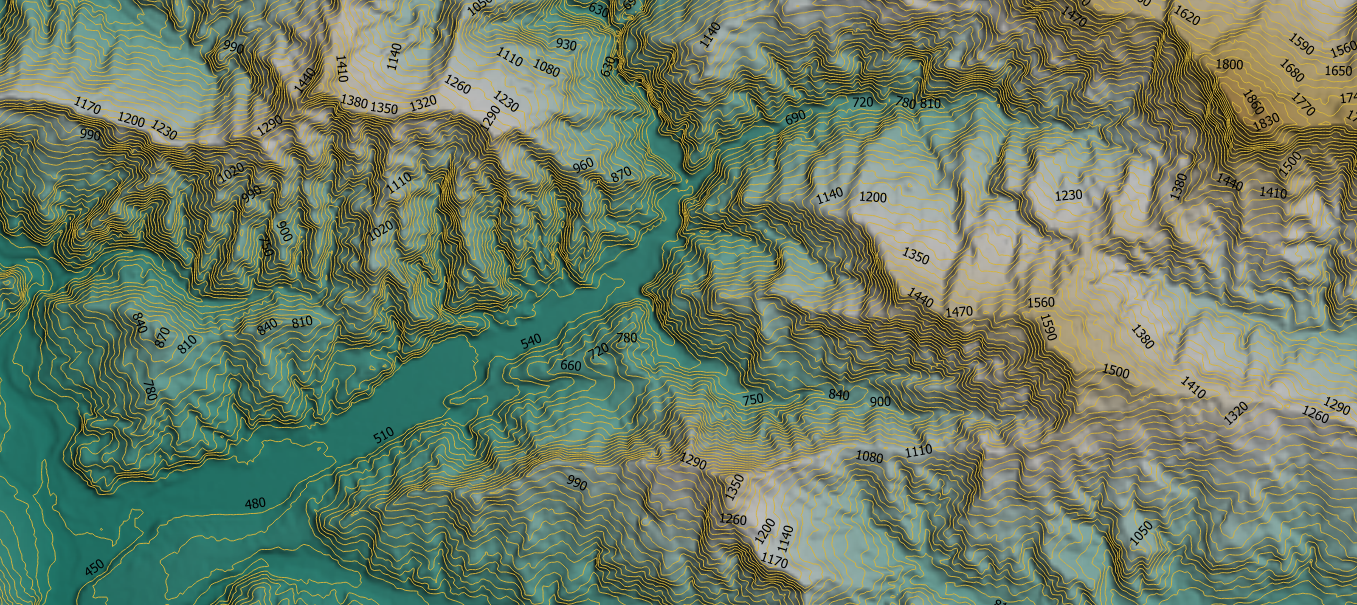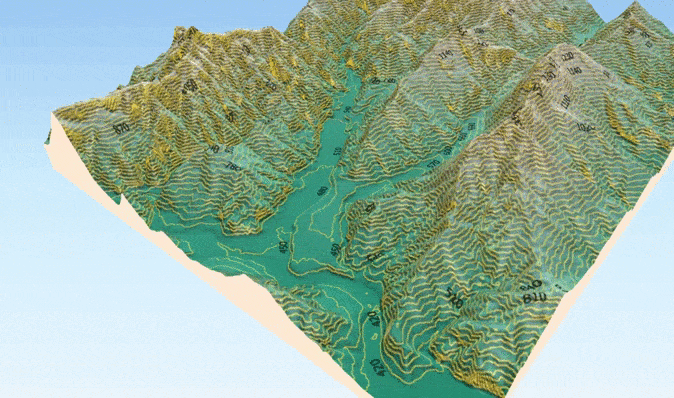# Creating contour lines from STRM and ALOS DEMs on Google Earth Engine

“Contour lines are an important way to show the rise and fall of the terrain on a map. They show all places that are at the same height above sea level. They also tell us about the slope of the terrain and are a layer information base in engineering projects “Contour lines generated from 30 m ALOS PALSAR DEM (Jaxa, 2020) on Google Earth Engine and QGIS platform in a central area of NEPALContour lines generated from the 30 m ALOS PALSAR DEM (Jaxa, 2020) on Google Earth Engine in NEPAL

# Basic creation: Option 1

`var dataset = ee.ImageCollection(‘JAXA/ALOS/AW3D30/V3_2’);var elevation = dataset.select(‘DSM’);// projectionvar proj = elevation.first().select(0).projection();var slopeReprojected = (elevation.mosaic().setDefaultProjection(proj));`
`// Remap values.var demclass = ee.Image(1)          .where(dem.gt(0).and(dem.lte(500)), 2)          .where(dem.gt(500).and(dem.lte(600)), 3)          .where(dem.gt(600).and(dem.lte(700)), 4)          .where(dem.gt(700).and(dem.lte(800)), 5)          .where(dem.gt(800).and(dem.lte(900)), 6)          .where(dem.gt(900).and(dem.lte(1000)), 7)          .where(dem.gt(1000).and(dem.lte(1100)), 8)          .where(dem.gt(1100).and(dem.lte(1200)), 9)          .where(dem.gt(1200).and(dem.lte(1300)), 10)          .where(dem.gt(1300).and(dem.lte(1400)), 11)          .where(dem.gt(1400).and(dem.lte(1500)), 12)          .where(dem.gt(1500).and(dem.lte(1600)), 13)          .where(dem.gt(1600).and(dem.lte(1700)), 14)          .where(dem.gt(1700).and(dem.lte(1800)), 15)          .where(dem.gt(1800).and(dem.lte(1900)), 16)          .where(dem.gt(1900).and(dem.lte(2000)), 17);          Map.addLayer(demclass.clip(AOI), {min: 1, max: 17, palette: ['black', 'white']}, 'elevationclass ALOS', false);`
`var vectors = zones.addBands(demclass).reduceToVectors({  geometry: AOI,  scale: 30,  geometryType: 'polygon',  eightConnected: false,  labelProperty: 'zone',  reducer: ee.Reducer.mean()});// Add the vectors to the mapvar display = ee.Image(0).updateMask(0).paint(vectors, '000000', 3);Map.addLayer(display, {palette: 'ff0000'}, 'contour lines ALOS', false);`

# Option 2

`var lines = ee.List.sequence(0, 4000, 100);`
`var contourlines = lines.map(function(line) {  var mycontour = strmelevation    .convolve(ee.Kernel.gaussian(5, 3))    .subtract(ee.Image.constant(line)).zeroCrossing()     .multiply(ee.Image.constant(line)).toFloat();      return mycontour.mask(mycontour);});contourlines = ee.ImageCollection(contourlines).mosaic();`
`Export.table.toDrive({  collection: vectors2,  description:'contour lines ALOS',  fileFormat: 'KML'});`